• 数学计算
千次阅读
2020-10-14 12:02:47

用c++进行数学计算
让用户输入一个圆的半径，计算这个院的周长和面积，并输出（保留两位小数）
#include
#include<Windows.h>

using namespace std;

int main(void){
float r;
float len;
float s;

cout<<"请输入半径：";
cin>>r;

len = 2 * 3.14 * r;
s = 3.14 * r *r;

cout.precision(2);
cout.flags(cout.fixed);

cout<<"周长是:"<<len<<endl;
cout<<"面积是"<< s <<endl;

system("pause");
return 0;


}c++
更多相关内容
• 初一数学计算题/专项练习、可以供计算能力差的学生使用，也可以供教师布置作业使用，以及培训机构出专项练习题，做专门训练
• 数学计算游戏.rar数学计算游戏.rar数学计算游戏.rar数学计算游戏.rar数学计算游戏.rar数学计算游戏.rarJavascript
• 易语言源码易语言数学计算汇编命令模块源码.rar 易语言源码易语言数学计算汇编命令模块源码.rar 易语言源码易语言数学计算汇编命令模块源码.rar 易语言源码易语言数学计算汇编命令模块源码.rar 易语言源码易语言...
• Scipy介绍 scipy是一个python开源的数学计算库，可以应用于数学、科学以及工程领域，它是基于numpy的科学计算库。 主要包含了统计学、最优化、线性代数、积分、傅里叶变换、信号处理和图像处理以及常微分方程的求解...

### Scipy介绍scipy是一个python开源的数学计算库，可以应用于数学、科学以及工程领域，它是基于numpy的科学计算库。

主要包含了统计学最优化线性代数积分傅里叶变换信号处理图像处理以及常微分方程的求解以及其他科学工程中所用到的计算

### scipy模块介绍

scipy主要通过下面这些包来实现数学算法科学计算，后面对于scipy的讲解主要也是基于这些包来实现的

• cluster：包含聚类算法
• constants：物理和数学上的一些常数
• fftpack：快速傅里叶变换
• integrate：积分和常微分方程的求解
• interpolate：插值和平滑的样条函数
• io：输入和输出
• linalg：线性代数
• ndimage：N维的图像处理
• ord：回归正交距离
• optimize：优化和寻根方程
• signal：信号处理
• sparse：稀疏矩阵
• spatial：空间数据结构和算法
• special：特殊的函数
• stats：统计分布和函数

### scipy的相关资料

展开全文scipy Python
• ## 用Python做数学计算之基础计算

万次阅读 多人点赞 2019-06-01 22:18:14
本文介绍使用Python来做数学计算，在学习和工作中，经常会遇到一些数学计算的问题。一般人会使用计算器软件，不得不说，计算器太难用了。专业人士可能会使用更强大的工具，如Matlab，但这种重量级工具有时可能并不...

原文链接: http://zh.5long.me/2015/python-math-1/

摘要（Abstract）

本文介绍使用Python来做数学计算，在学习和工作中，经常会遇到一些数学计算的问题。一般人会使用计算器软件，不得不说，计算器太难用了。专业人士可能会使用更强大的工具，如Matlab，但这种重量级工具有时可能并不适用。本文打算使用一个轻量级的工具Python来做计算。准确来说Python并不是一个数学工具，而是一种编程语言。Python提供了很多数学库，利用Python和这些库可以做很多数学计算。

本文不是编程语言的教程，更像是一个工具的使用教程，阅读本文不需要有程序设计基础，当然，需要一点数学基础（比如加减乘除）。本文适合任何想找一个计算工具的人学习和参考。

本文将以实例讲解各种用法。

安装Python（Installation）

提示1：Mac OS和大部分版本Linux系统自带Python运行环境，可以不用安装。当然，也可升级成最新版本。Windows需要自行安装。
提示2：也可以安装Sublime Text编辑器，再安装Sublime REPL插件。本人现在使用这种方案，写Python程序非常方便。

提示3：搜狗输入法用户注意，搜狗输入法在IDLE下有点小问题（不能退格），切换到英文输入法即可。

Python 2 和Python 3的注意事项

print的语法。python 3的用法为print("hello world!")，python 2的用法为print "hello world!"或者print("hello world!")。

基本运算

加法

>>> 1 +  2      //直接输入，回车直接输出结果
3
>>> sum = 1 + 2 //计算结果保存在sum中
>>> print(sum)      //输出sum
3
>>> a = 1       //变量
>>> b = 2
>>> sum = a + b     //变量相加
>>> print(sum)
312345678910

减法

>>> a = 1
>>> b = 2
>>> 2 - 1
1
>>> a - b
-1
>>> b - a
112345678

乘法

>>> 1 * 2
2
>>> 1.5 * 3
4.5
>>> a * b
2
>>> 1234567

除法

传统的除法。有编程经验的人一定知道，整型除法的结果也是整型（地板除，舍弃小数部分）。如果有一个数是浮点型，则结果是浮点型（真正的除法，保留小数）。

>>> 1 / 3           //整型除法，商为0，舍弃小数部分
0
>>> 5 / 2
2
>>> 1.0 / 3         //浮点数除法，保留小数
0.3333333333333333
>>> 5.0 / 2.0
2.512345678

真正的除法。在未来的Python版本中，不管是整型还是浮点型除法，结果总是真实地商（保留小数部分），现阶段可以通过执行from __future__ import division指令做到这一点。

>>> from __future__ import division
>>> 1 / 3
0.3333333333333333
>>> 5 / 2
2.5
>>> 1.0 / 3
0.3333333333333333
>>> 5.0 / 2.0
2.5123456789

地板除。Python 2.2新加了一个操作符//，//除法不管操作数为何种数值类型，总是舍弃小数部分。

>>> 1 // 3
0
>>> 5 // 2
2
>>> 1.0 // 3
0.0
>>> 5.0 // 2.0
2.012345678

取余数

>>> 1 % 3
1
>>> 5 % 2
1
>>> 5.0 % 2.0
1.0123456

幂运算

Python有幂运算符**。

>>> 2 ** 3
8
>>> 2.5 ** 5
97.65625
>>> 4 ** -1
0.25123456

代码中分别计算23、2.55、4-1。

复数

复数的表示。复数的表示如下：

>>> aComplex = 1 + 2j       //申明一个复数
>>> aComplex
(1+2j)
>>> aComplex.real           //复数实部
1.0
>>> aComplex.imag           //复数虚部
2.0
>>> aComplex.conjugate()        //共轭复数
(1-2j)
>>> 12345678910

复数的运算。复数的运算与实数一致。

>>> c = 1 + 2j
>>> d = 2 - 1j
>>> c + d
(3+1j)
>>> c - d
(-1+3j)
>>> c * d
(4+3j)
>>> c / d
1j
>>> c / 2
(0.5+1j)
>>> c * 2
(2+4j)
>>> c ** 2
(-3+4j)12345678910111213141516

math标准库

Python有一个标准库math专门用来做数学运算的。详细介绍可参考Python的官方文档。要使用math库，先要import这个库。

>>> import math1

一下的例子假设已经执行了import math。

两个常数

>>> math.pi             //圆周率pi
3.141592653589793
>>> math.e
2.718281828459045       //自然常数e1234

数值计算

math.ceil(x)。向上取整，返回最小的大于或等于x的整数。

>>> math.ceil(2)
2.0
>>> math.ceil(2.2)
3.0
>>> math.ceil(2.9)
3.0
>>> math.ceil(3.0)
3.012345678

math.floor(x)。向下取整，返回最大的小于或等于x的整数。

>>> math.floor(2)
2.0
>>> math.floor(2.2)
2.0
>>> math.floor(2.9)
2.0
>>> math.floor(3.0)
3.012345678

math.fabs(x)。取x得绝对值。

>>> math.fabs(1.0)
1.0
>>> math.fabs(-1.0)
1.01234

math.factorial(x)。求x的阶乘，x必须为整数，否则出现错误。

>>> math.factorial(5)
120
>>> math.factorial(4)
24
>>> math.factorial(2.1)     //执行错误
Traceback (most recent call last):
File "<stdin>", line 1, in <module>
ValueError: factorial() only accepts integral values
>>> 123456789

幂和对数函数（Power and logarithmic functions）

math.exp(x)。返回e ** x。

>>> math.exp(2)
7.38905609893065
>>> math.e ** 2
7.3890560989306495  //请忽略后面的不一致，计算机浮点数本身的问题1234

math.log(x [,base])。求以base为底的对数。

>>> math.log(math.e)        //值传一个参数，默认以math.e为底
1.0
>>> math.log(math.e ** 2)
2.0
>>> math.log(8, 2)      //两个参数，2为底
3.0
>>> math.log(100, 10)   //两个参数，10为底s
2.012345678

math.pow(x, y)。幂运算，计算xy，相当于x ** y。

>>> math.pow(2, 3)
8.0
>>> 2 ** 3
81234

math.sqrt(x)**。求x的平方根。

>>> math.sqrt(4)
2.0
>>> math.sqrt(9.0)
3.01234

开根号。Python的math库中只有开平方根，没有立方根和n次方根，不过可以利用math.pow或者**，只需把根号变成分数。

>>> math.pow(4, 1.0 / 2)        //平方根，相当于math.sqrt(4)
2.0
>>> 4 ** (1.0 / 2)          //平方根，相当于math.sqrt(4)
2.0
>>> 8 ** (1.0 / 3)          //立方根
2.0
>>> 1024 ** (1.0 / 10)          //10次方根
2.012345678

三角函数（Trigonometric functions）

方法名
实现功能

math.degrees(x)
把弧度x转换成角度

把角度x转换成弧度

>>> math.degrees(math.pi)
180.0
3.1415926535897931234

math.cos(x)。不解释。
math.sin(x)。不解释。
math.tan(x)。不解释。
math.acos(x)。反余弦函数。
math.asin(x)。反正弦函数。
math.atan(x)。反正切函数。
math.hypot(x，y)。欧式范数（Euclidean norm）。相当sqrt(x*x + y*y)。

>>> math.cos(math.pi)
-1.0
>>> math.sin(math.pi / 2)
1.0
>>> math.tan(math.pi / 4)
0.9999999999999999      //结果为1，计算机浮点数固有缺陷
>>> math.tan(math.pi / 4)
0.9999999999999999
>>> math.acos(-1.0)
3.141592653589793
>>> math.asin(1.0)
1.5707963267948966
>>> math.atan(1)
0.7853981633974483
>>> math.pi / 4
0.7853981633974483
>>> math.hypot(3, 4)
5.0123456789101112131415161718

双曲函数（Hyperbolic functions）

双曲函数和三角函数类似。

math.cosh(x)。求x的双曲余弦函数。
math.sinh(x)。求x的双曲正弦函数。
math.tanh(x)。求x的双曲正切函数。
math.acosh(x)。求x的反双曲余弦。
math.asinh(x)。求x的反双曲正弦。
math.atanh(x)。求x的反双曲正切。

>>> math.cosh(1)
1.5430806348152437
>>> math.sinh(1)
1.1752011936438014
>>> math.tanh(1)
0.7615941559557649
>>> math.acosh(1.5430806348152437)
1.0
>>> math.asinh(1.1752011936438014)
1.0
>>> math.atanh(0.7615941559557649)
0.9999999999999999          //结果为1，计算机浮点数固有缺陷123456789101112

一个复杂的例子

举一个复杂的例子，计算下面的公式：

>>> - (4 ** (1.0 / 3)) / 4 * math.log(2 ** (1.0 / 3) - 1) - (math.sqrt(3) * (4 ** (1.0 / 3))) / 6 * math.atan(math.sqrt(3) / (1 + 2 * (2 * (1.0 / 3))))
0.2420914086273389712

在前面的例子可能已经注意到了，计算机表示浮点数是不准确的，比如1.0成了0.9999999999999999。这是计算机浮点数表示法的固有缺陷。比如会出现如下结果：

>>> 0.05 + 0.01
0.060000000000000005
>>> 1.0 - 0.12
0.88
>>> 1.0 - 0.32
0.6799999999999999
>>> 1.0 - 0.42
0.5800000000000001
>>> 4.015 * 100
401.49999999999994
>>> 123.3 / 100
1.2329999999999999123456789101112

浮点数的缺陷不仅仅在Python在存在，在任何语言都存在，它是浮点数表示法本身的缺陷。对于不是非常严格的计算，这种缺陷是可以接受的，而对于过于严格的计算，那么应该用整型数计算（整型计算是精确的）。比如在保留两位小数的计算中，可以把原始数乘以100取整数再计算，约定最后两位为小数即可。

结语

本文介绍了Python标准库的数学计算方法，通过使用Python标准数学库，可以应付一些日常的基本计算（比如我就很少使用计算器了，一般的计算都用Python解决）。当然，数学是如此的丰富，仅有标准库是远远不够的。将来如果有需要的话，我会研究下使用其它的数学库来进行更复杂的计算以及数学图像的绘制。比如NumPy、SciPy、Matplotlib、SymPy等。

# 函数定义

在python中函数的定义以及调用如下代码所示：

def test(x):
y = x+1
return y

result = test(2)
print(result)

# 多个返回值的情况

如果在函数中return多个值，会将那多个值打包成一个元组传出，如下代码所示def test(x):
y1 = x+1
y2 = x+2
return y1,y2

result = test(2)
print(result) #打印结果为(3, 4)# 使用关键字参数的情况

使用关键字参数，则传参的位置可以不固定，但是个数还是要相匹配，此外在调用的时候关键字参数一定要在普通参数的右边，如下代码所示

def test(x,y,z):
res = x - y - z
return res

result = test(y=1,x=5,z=2)
print(result) #打印结果为2

# 使用默认参数的情况

使用默认参数，传参的个数可以不匹配，如果默认参数的位置没有传，则使用默认值，这个是在定义的时候定义的，定义默认参数要写在最右边，如下代码所示def test(name,sex = "man"):
print(name)
print(sex)

test("CodeScrew")
#打印结果为CodeScrew  man
test("CodeScrew","women")
#打印结果为CodeScrew  women# 使用参数组的情况

参数组中指的是可以传任意参数，一般**对应字典，*对应列表，如下代码所示

• 传入列表
def test(name,*args):
print(name)
print(args)

test("CodeScrew",*[1,2,3,4])
#打印结果为CodeScrew   (1, 2, 3, 4)
• 传入字典def test(name,**kwargs):
print(name)
print(kwargs)

test("CodeScrew",x=1,y=2)
#打印结果为CodeScrew   {'x': 1, 'y': 2}
test("CodeScrew",**{"x":1,"y":2})
#打印结果为CodeScrew   {'x': 1, 'y': 2}def test(x,y,z):
res = x - y - z
return res

result = test(y=1,x=5,z=2)
print(result) #打印结果为2

方法一: 使用内置模块

>>> import math >>> math.pow(12, 2)     # 求平方144.0 >>> math.sqrt(144)      # 求平方根12.0 >>>

方法二: 使用表达式

>>> 12 ** 2             # 求平方144 >>> 144 ** 0.5          # 求平方根12.0 >>>

方法三: 使用内置函数

>>> pow(12, 2)          # 求平方144 >>> pow(144, .5)        # 求平方根12.0

展开全文• 本次是《图解Pandas》系列图文内容的 第 06 篇，主要介绍 Pandas 中常见的数学计算 。本文是付费阅读文章，付费阅读内容包括《图解Pandas》图文干货内容、可以在电脑端观看的视频以及配套的源代码等。此外，为避免...

## 01写在前面

大家好，我是阳哥，欢迎来到「Python数据之道」。

本次是《图解Pandas》系列图文内容的 第 06 篇，主要介绍 Pandas 中常见的数学计算

本文是付费阅读文章，付费阅读内容包括《图解Pandas》图文干货内容、可以在电脑端观看的视频以及配套的源代码等。

此外，为避免各种不必要的麻烦，微信没有退款机制，付费前请谨慎。

Pandas 是 Python数据分析中用的最多的工具，为了大家能够更好地学习 Pandas，阳哥开始制作一系列 《图解Pandas》 的内容。

《图解Pandas》 最主要的目标，是以动态图片、视频等方式呈现，拆解 Pandas 在数据处理过程中的主要知识点，以便大家能进一步了解 Pandas 的使用，形象地介绍 Pandas，进而使大家喜欢上 Pandas 这个得心应手的工具。

《图解Pandas》图文专题，最新的内容可以点击下面的图片进行查看。在本文编写时，《图解Pandas》系列已发布的图文如下：

本次主要介绍 Pandas 中常见的数学计算 ，内容框架如下：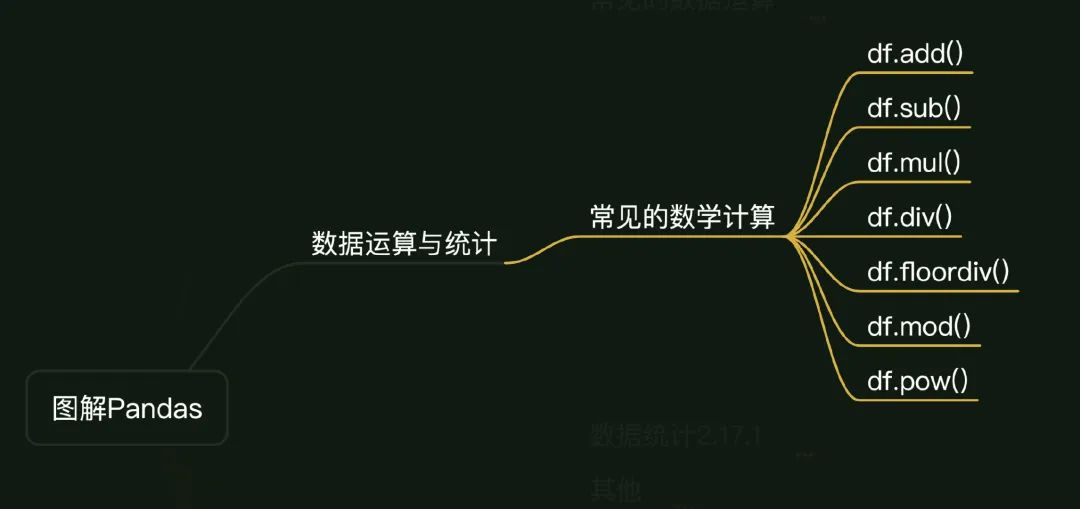在微信视频号「Python数据之道」中发布的对应视频编号为 009010，大家可以前往观看：

扫描下面的二维码，关注视频号，可以观看《图解Pandas》已发布的所有视频以及及时推送最新的视频：### Python环境

开始之前，按照惯例先介绍下本次内容涉及的Python环境，如下：

• Python 3.8

• Pandas 1.4.1

• numpy 1.22.3

我是在 jupyter notebook 中运行代码的，首先引入相关 Python 库：

import pandas as pd
import numpy as np

print(f'pandas version:{pd.__version__}')
print(f'numpy version:{np.__version__}')

# pandas version:1.4.1
# numpy version:1.22.3

## 02常见的数学计算

### 创建数据

咱们先来创建一个dataframe，也就是“数据框”。

关于创建数据对象的详细介绍，大家可以观看 「图解Pandas」系列的第2期视频

创建 df 如下：

d = {
"name": [ "Lemon", "Jack", "Peter","Emma","James"],
"city": ["长沙", "上海", "深圳","北京","杭州"],
"a": [80, 90, 60,73,89],
"b": [80, 75, 80,85,83],
"c": [70, 75, 80,73,62],
}
df = pd.DataFrame(d)
df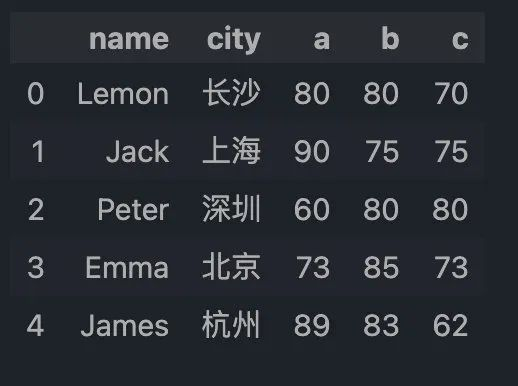来看看数据框 df 的数据结构，为 5 行 5 列，数值类型主要是字符串和整型。后续，还会在该数据框的基础上衍生出其他的一些数据框。

Pandas中常见的数学计算，主要包括加、减、乘、除、整除、取余、乘方等几种。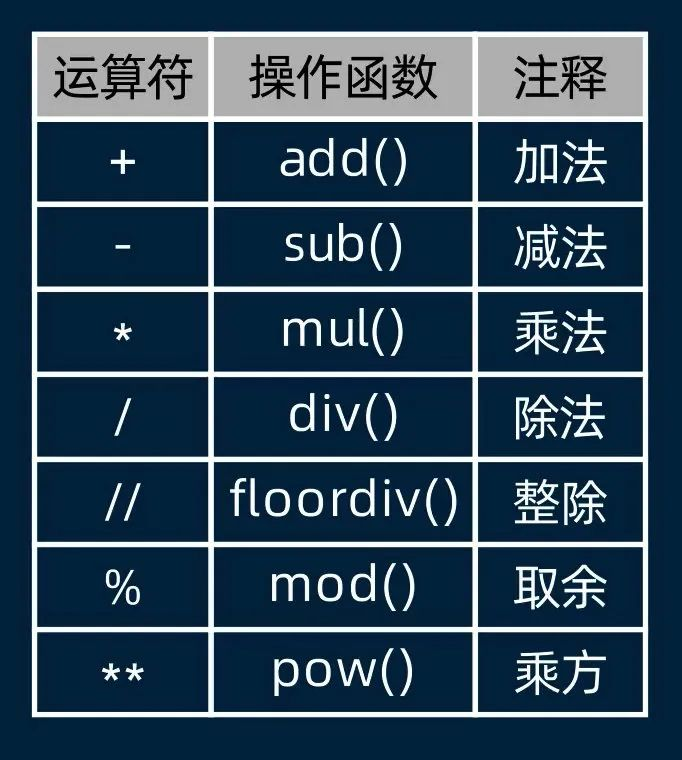后面将从四个方面来对这些数学计算方式展开描述，包括:

• 列与标量的数学计算

• 列与列的数学计算

• 逆方向操作

• 案例实践

### 列与标量的数学计算

一般情况下，数学计算，需要数据框中的数值类型为整型、浮点数等数字类型。

先来介绍列与标量的数学计算，下面这个是加法的案例，直接用 + 就可以。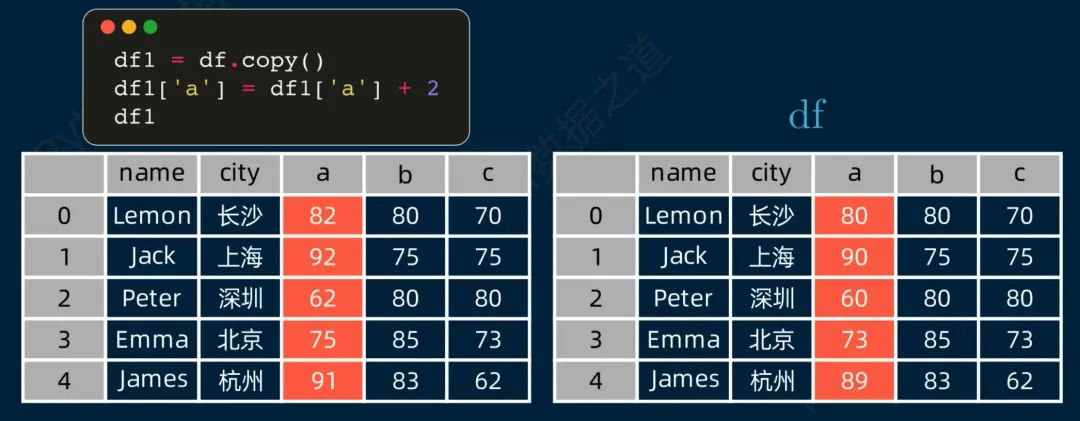数据框 “df” 的 a 列加 2，相当于 a 列的每个元素都加上 2，其结果在数据框 “df1” 中体现。也可以用 add() 函数来进行加法计算，其结果是一样的，如下：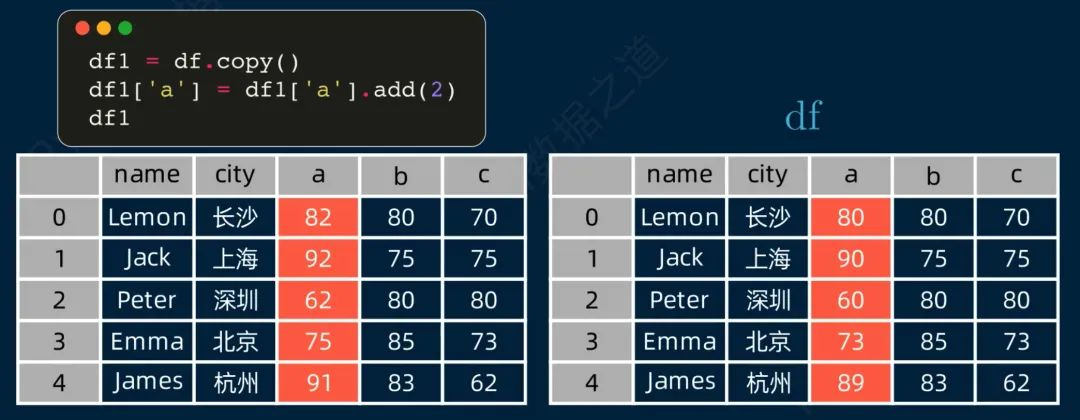使用 add() 函数与加法的数学符号是稍微有些不一样的地方的，主要是使用函数可以设置一些参数，比如当数据中有 NA 值时，就可以通过设置参数 fill_value 来计算。

展开全文• 浅析小学学生数学计算能力的培养 　[导读] 本文是小学数学优秀论文范文2000字至3000字完整版《新课程标准》提出了关于“使学生能够正确地进行整数、小数、分数的四则计算，对其中一些基本的计算，要达到一定的熟练...
• 小学三年级数学计算题自动出题器1.01 出计算题是一件麻烦事 有了这个出题器 一点就搞定!
• package main import ( "fmt" "github.com/robaho/fixed" ) func main() { price, err := fixed.NewSErr("136.02") if err != nil { panic(err) } quantity := fixed.NewF(3)... fee, _ := fixed.NewSErr...
• package AL.java; public class MyMath { public static int abs(int x) { if(x < 0) return -x;... //计算绝对值 public static double abs(double x) { if(x < 0.0) return -x...java
• ## MATLAB基础学习（一）基本数学计算

万次阅读 多人点赞 2019-01-09 15:27:35
基本计算 MATLAB中的基本的运算符号为： + - * / ^ 四则运算规则和平时使用的计算器相同，使用MATLAB进行计算时也需要注意计算的顺序，先加减后乘除，需要改顺序的时候需要使用括号。 接下来是通过具体的例子...MATLAB
• ## shell中的数学计算

千次阅读 2018-06-30 15:30:00
shell中的赋值和操作默认都是字符串处理，在此记下shell中进行数学运算的几个特殊方法，以后用到的时候可以来看，呵呵。 1、错误方法举例 a) var=1+1 echo $var 输出的结果是1+1，悲剧，呵呵 b) var=1 var=$...
• Math.NET的目标是为提供一款自身包含清晰框架的符号运算和数学运算/科学运算，它是C#开发的开源类库。Math.NET含了一个支持线性代数的解析器，分析复杂微分，解方程等等功能。这个项目大部分采用的是MIT/X11开源软件...
• 数值计算 ndarray、矩阵 Scipy 科学计算 傅立叶变换、信号处理、稀疏矩阵 Matplotlib 画图 plot、scatter、bar Pandas 数据分析 Series、DataFrame Scikit-learn 机器...
• 是一个用于计算复杂数学表达式并能够图形化显示计算结果的Java开源类库。它是Matlab、Octave、FreeMat、Scilab的一个克隆，但完全采用纯Java实现。 4. work上推荐  SCaVis  实现了科学计算、数据分析和数据可视...
• ## Linux中的数学计算

千次阅读 2018-11-10 16:05:12
第一次使用Shell中的数学运算的时候，相对于C/C++中的直接使用来说，十分的麻烦，并且不是很好记忆 expr方法 expr方法可以直接在命令行使用，当遇到在Shell中有其他含义的操作符的时候，需要使用'\'转义符，并且...expr Linux shell
• npm install number-precision --save import NP from 'number-precision' NP.strip(0.09999999999999998); // = 0.1 NP.plus(0.1, 0.2); // = 0.3, not 0.30000000000000004 NP.plus(2.3, 2.4);...
• 一、符号数学计算（Maple） 1、符号对象的创建 %符号变量的创建 clc;clear; syms x y f(x,y) %多个符号变量sym要加s f(x,y) = x + 2 * y %在命令行窗口中如果直接输入一个没有进行初始化的变量，会出现无法识别的...matlab
• VB 用数组实现数学计算 VB 用数组实现数学计算
• ## R语言中的数学计算（转载）

万次阅读 多人点赞 2016-06-07 23:04:34
R语言中的数学计算关于作者：张丹(Conan), 程序员Java,R,PHP,Javascript weibo：@Conan_Z blog: http://blog.fens.me email: bsspirit@gmail.com 转载请注明出处： http://blog.fens.me/r-mathematics/r-math...R
• 编写程序，提供实现各种数学计算的方法。 包括如下几项： （1）两个数的加、减、乘、除。 （2）求某数的相反数、倒数、绝对值。 （3）取两数中较大的和较小的。 方法一：定义常量 方法二：给方法传值 ...
• 输入一个数学计算表达式，如:1+2 然后输出计算后的结果:1+2=3 这里计算表达式只计算一次即可，可以使用加减乘除任意一个,可以进行小数运算。 public class Test07 { public static void main(String[] args) { ...
• 数学计算手抄报的图片欣赏数学计算手抄报图一数学计算手抄报图二数学计算手抄报图三数学计算手抄报图四数学计算手抄报图五数学计算手抄报的资料一：数学图形计算公式1、正方形 (C：周长 S：面积 a：边长)周长=边长×...
• 在编写程序过程中，常常用到数学计算方法。C++提供简单公式的计算方法。但是在编写程序过程中要注意头文件cmath.h或math.h。常用的方法如下所示： 一、幂计算 开方：double sqrt(double x); x的y次方：double pow...C++ 函数
• 软件为小学数学计算训练软件，涵盖多种小学数学计算题类型，非常适合家长和老师对小孩进行计算训练或竞赛使用。 链接：https://pan.baidu.com/s/1LKYeA80gW8V9xn4WL2s_Lg 提取码：03z9 支持选择范围，选择计算方式...
• http://www.yunsuanzi.com/matrixcomputations/index.html
• Prolog中也有一些能够进行数学计算的功能，但是数学计算是不好用逻辑的事物来描述的。因此计算一个数学表达式的方法和我们以前所学习的模式匹配有很大的区别。因此，Prolog专门提供了内部谓词is来计算数学表达式。其......